Question

# An earth satellite moves in a circular orbit with an orbital speed of 5800 m/s

An earth satellite moves in a circular orbit with an orbital speed of 5800 m/s.

Find the time (expressed in seconds) of one revolution of the satellite.

Find the radial acceleration of the satellite in its orbit.

I think we need to know the radius of the earth and the satellite's average distance from the earth in order to answer the question. All we have right now is the angular velocity. If you have that information, then use
d=v*t where d is the diameter=pi*2*r and
a=v^2/r is the angular acceleration.

The length of time it takes for a satellite to orbit the earth, its orbital period, varies with the altitude of the satellite above the earth's surface. The lower the altitude, the shorter the period. The higher the altitude, the longer the period. For example, the orbital period for a 100 mile high satellite is ~88 minutes; 500 miles ~101 minutes; 1000 miles ~118 minutes; 10,000 miles 9hr-18min; 22,238 miles 23hr-56min-4.09sec. A satellite in an equatorial orbit of 22,238 miles altitude remains stationary over a point on the Earth's equator and the orbit is called a geostationary orbit. A satellite at the same 22,238 miles altitude, but with its orbit inclined to the equator, has the same orbital period and is referred to as a geosynchronous orbit as it is in sync with the earth's rotation.
Not surprisingly, the velocity of a satellite reduces as the altitude increases. The velocities at the same altitudes described above are 25,616 fps. (17,426 mph) for 100 miles, 24,441 fps. (16,660 mph.) for 500 miles, 23,177 fps. (15,800 mph.) for 1000 miles, 13,818 fps. (9419 mph) for 10,000 miles, and 10,088 fps. (6877 mph.) for 22,238 miles.

Depending on your math knowledge, you can calculate the orbital velocity and orbital period from two simple expressions. You might like to try them out if you have a calculator.
The time it takes a satellite to orbit the earth, its orbital period, can be calculated from

T = 2(Pi)sqrt[a^3/µ]

where T is the orbital period in seconds, Pi = 3.1416, a = the semi-major axis of an elliptical orbit = (rp+ra)/2 where rp = the perigee (closest) radius and ra = the apogee (farthest) radius from the center of the earth, µ = the earth's gravitational constant = 1.407974x10^16 ft.^3/sec.^2. In the case of a circular orbit, a = r, the radius of the orbit. Thus, for a 250 miles high circular orbit, a = r = (3963 + 250)5280 ft. and T = 2(3.1416)sqrt[[[(3963+250)5280]^3]/1.407974x10^16] = ~5555 seconds = ~92.6 minutes.

The velocity required to maintain a circular orbit around the Earth may be computed from the following:

Vc = sqrt(µ/r)

where Vc is the circular orbital velocity in feet per second, µ (pronounced meuw as opposed to meow) is the gravitational constant of the earth, ~1.40766x10^16 ft.^3/sec.^2, and r is the distance from the center of the earth to the altitude in question in feet. Using 3963 miles for the radius of the earth, the orbital velocity required for a 250 miles high circular orbit would be Vc = 1.40766x10^16/[(3963+250)x5280] = 1.40766x10^16/22,244,640 = 25,155 fps. (17,147 mph.) Since velocity is inversely proportional to r, the higher you go, the smaller the required orbital velocity.

The question here is circumspect: As TchrWill points out, orbital velocity depends on radius. The slower it goes, the higher it is.

Centripetal acceleration= V^2/r
setting these equal...

v^2/r= g re^2/r^2
or r= g re^2/v^2

So period= 2PI r/V= 2PI g re^2/v^3

T= 2*3.14g*(6.38E6)^2 /(5800)^3

You do it. I get over three hours, at a very high altitude.

Hi! Very nice site! Thanks you very much! 7wts3vjdwJo

Hello! Good Site! Thanks you! bizbldoouex

We need at least 10 more requests to produce the answer.

0 / 10 have requested this problem solution

The more requests, the faster the answer.

All students who have requested the answer will be notified once they are available.

#### Earn Coins

Coins can be redeemed for fabulous gifts.

Similar Homework Help Questions
• ### The time in hours it takes a satellite to complete an orbit around the earth varies directly as the radius of the orbit (from the center of the earth) and inversely as the orbital velocity

The time in hours it takes a satellite to complete an orbit around the earth varies directly as the radius of the orbit (from the center of the earth) and inversely as the orbital velocity. If a satellite completes an orbit 860 miles above the earth in 12 hours at a velocity of 34,000 mph, how long would it take a satellite to complete an orbit if it is at 1700 miles above the earth at a velocity of 27,000...

• ### A spy satellite is in circular orbit around Earth

A spy satellite is in circular orbit around Earth. It makes one revolution in 6.01 hours. (a) How high above Earth's surface is the satellite? [Answer in Km] (b) What is the satellite's acceleration? [Answer in m/s^2] I see this posted over and over again and I have about 4 equations, 20 different answers, and none are correct. NOTE: There is no radius or velocity available to enter into the equations. I have used T=2 pi sqrt(r^3/G) and the r...

• ### A satellite is in a circular orbit around the Earth at an altitude of 2.52 106...

A satellite is in a circular orbit around the Earth at an altitude of 2.52 106 m. (a) Find the period of the orbit. (Hint: Modify Kepler's third law:           T2 =(4π^2/GMs)r^3 so it is suitable for objects orbiting the Earth rather than the Sun. The radius of the Earth is 6.38 106 m, and the mass of the Earth is 5.98 1024 kg.) _______________h (b) Find the speed of the satellite. _________km/s (c) Find the acceleration of the satellite....

• ### A 544-kg satellite is in a circular orbit about Earth at a height above Earth equal...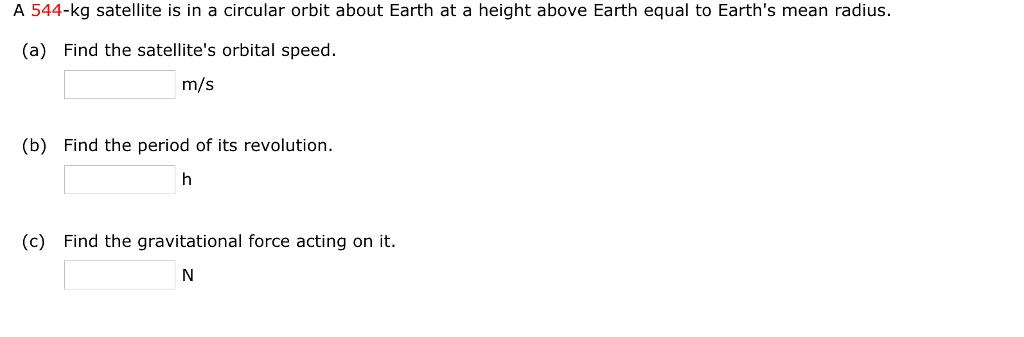A 544-kg satellite is in a circular orbit about Earth at a height above Earth equal to Earth's mean radius. (a) Find the satellite's orbital speed. m/s (b) Find the period of its revolution. (c) Find the gravitational force acting on it A satellite of Mars, called Phobos, has an orbital radius of 9.4 x 106 m and a period of 2.8 104 s. Assuming the orbit is circular, determine the mass of Mars. x 10 s. Assuming kg

• ### What is the orbital speed of a satelite in a circular orbit at 8, 129 kilometers...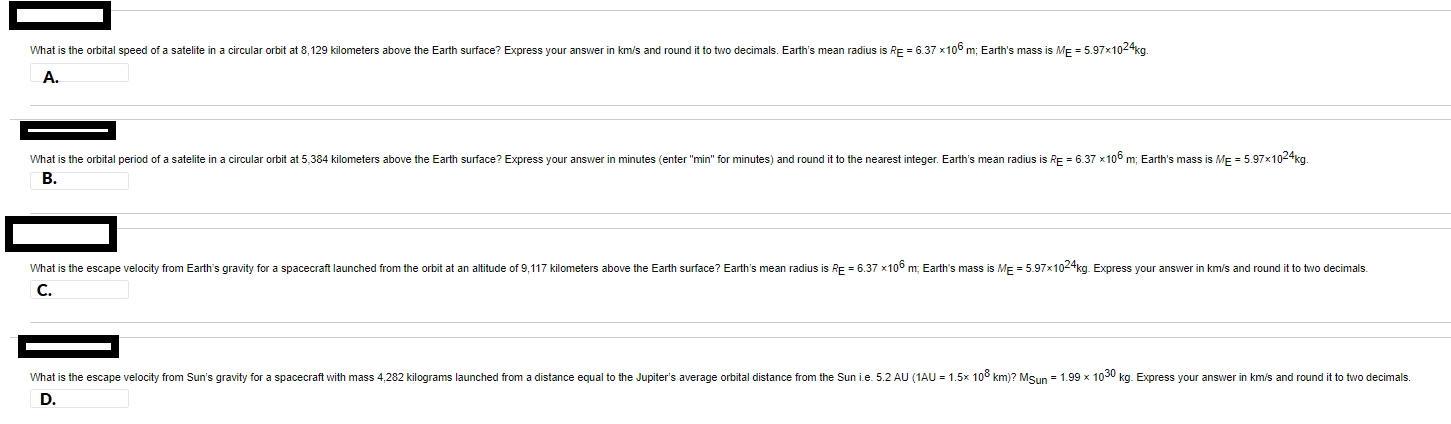What is the orbital speed of a satelite in a circular orbit at 8, 129 kilometers above the Earth surface? Express your answer in km/s and round it to two decimals. Earth's mean radius is RE = 6.37 x100 m; Earth's mass is ME = 5.97x104kg. A What is the orbital period of a satelite in a circular orbit at 5,384 kilometers above the Earth surface? Express your answer in minutes (enter "min" for minutes) and round it to the...

• ### Consider a satellite of mass m moving in a circular orbit around the Earth at a...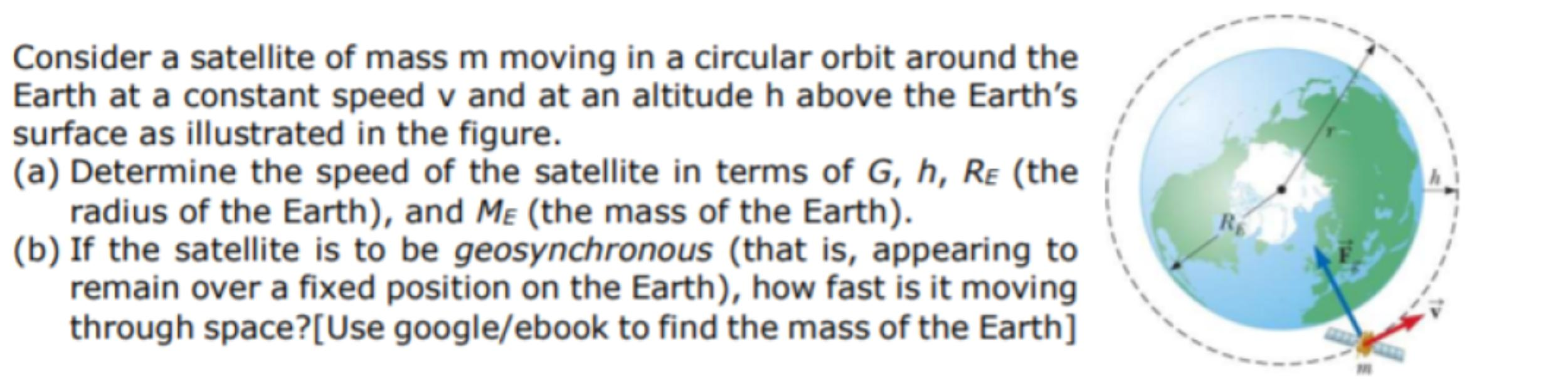Consider a satellite of mass m moving in a circular orbit around the Earth at a constant speed v and at an altitude h above the Earth's surface as illustrated in the figure. (a) Determine the speed of the satellite in terms of G, h, Re (the radius of the Earth), and Me (the mass of the Earth). (b) If the satellite is to be geosynchronous (that is, appearing to remain over a fixed position on the Earth), how fast...

• ### A satellite in a circular orbit 879.4 mi above the earth makes one complete orbit every 83.42min

A satellite in a circular orbit 879.4 mi above the earth makes one complete orbit every 83.42min. What is its linear velocity? Use 3963 mi for the length of the radius of the earth.

• ### 2. Consider a system consisting of the Sun, Earth, and a satellite in a circular orbit...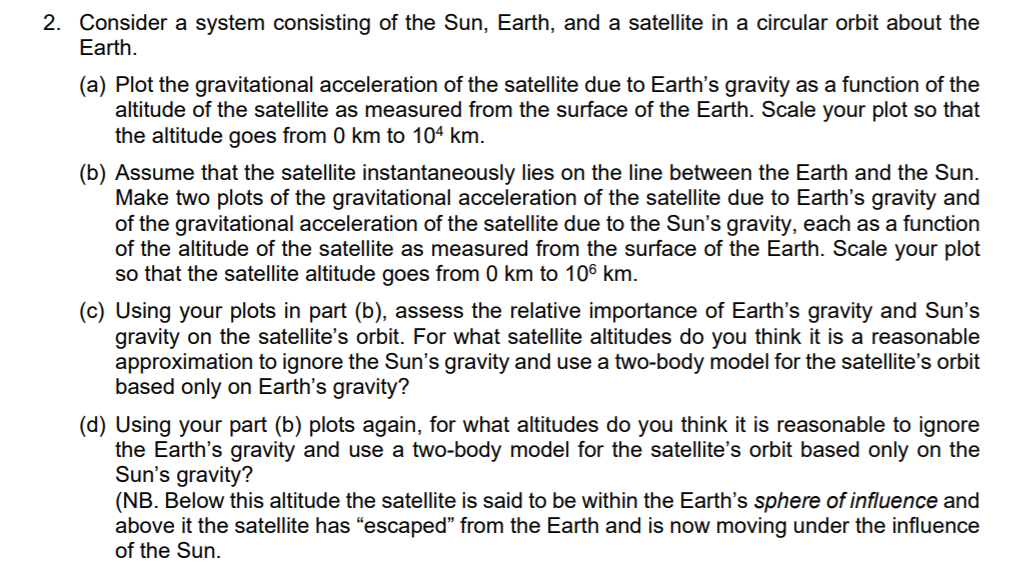2. Consider a system consisting of the Sun, Earth, and a satellite in a circular orbit about the Earth. (a) Plot the gravitational acceleration of the satellite due to Earth's gravity as a function of the altitude of the satellite as measured from the surface of the Earth. Scale your plot so that the altitude goes from 0 km to 104 km. (b) Assume that the satellite instantaneously lies on the line between the Earth and the Sun. Make two...

• ### 4. Consider a satellite of mass m moving in a circular orbit around the Earth at...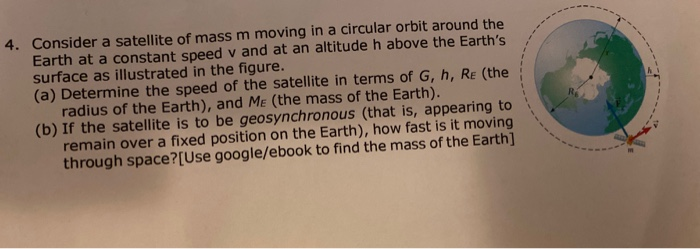4. Consider a satellite of mass m moving in a circular orbit around the Earth at a constant speed v and at an altitude h above the Earth's surface as illustrated in the figure. (a) Determine the speed of the satellite in terms of g, h, Re (the radius of the Earth), and Me (the mass of the Earth). (b) If the satellite is to be geosynchronous (that is, appearing to remain over a fixed position on the Earth), how...

• ### A spy satellite is in circular orbit around Earth

A spy satellite is in circular orbit around Earth. It makes one revolution in 6.05 hours. (Radius of the Earth=6.371 106 m) (a) How high above Earth's surface is the satellite? What is the satellite's acceleration